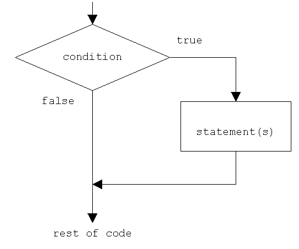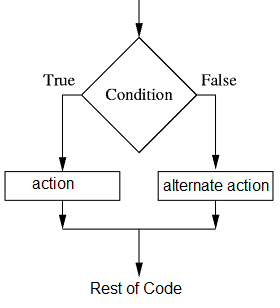Python Tutorial

# Python Conditional Statements

Decision-making stands as a cornerstone within the scope of computer programming, embodying paramount significance. It necessitates developers to carefully delineate one or more conditions, subject to evaluation or testing by the program. Linked with these conditions are statements, poised for execution contingent upon their truth. Moreover, provisions for alternative executions, under the aegis of falsehood, can also be optionally defined. The Python programming language offers an array of decision-making constructs, furnishing a versatile toolkit for this purpose.

1. if statements
2. if....else statements
3. if..elif..else statements
4. nested if statements
5. not operator in if statement
6. and operator in if statement
7. in operator in if statement

## Python if statements

if expression: statementsIn Python, the "if" statement assesses the test expression enclosed within parentheses. Should the test expression resolve to a true value (nonzero), the statements housed within the "if" block are executed. Conversely, if the test expression evaluates to a false value (0), the statements within the "if" block are bypassed.

example
x=20 y=10 if x > y : print(" X is bigger ")
output
X is bigger

Within this program, two variables, namely 'x' and 'y', are instantiated. 'x' is initialized with the value 20, and 'y' with 10. Subsequently, the "if" statement scrutinizes the expression (x > y) for its veracity. In this specific instance, the evaluation x > y holds true, since 'x' assumes the value 20 while 'y' is set at 10. As a consequence, the program's control is directed into the body of the "if" block, leading to the printing of the message "X is bigger." In scenarios where the condition proves to be false, the program's flow proceeds beyond the confines of the "if" block.

## Python if..else statements

The "else" statement serves the purpose of defining a code block that is to be executed when the condition within the "if" statement turns out to be false. As a result, the "else" clause guarantees the execution of a predetermined sequence of statements.if expression: statements else: statements
example
x=10 y=20 if x > y : print(" X is bigger ") else : print(" Y is bigger ")
output
Y is bigger

In the above code, the if stat evaluate the expression is true or false. In this case the x > y is false, then the control goes to the body of else block , so the program will execute the code inside else block.

## if..elif..else statements

if expression: statements elif expression: statements else: statements

The elif is short for else if and is useful to avoid excessive indentation.

example
x=500 if x > 500 : print(" X is greater than 500 ") elif x < 500 : print(" X is less than 500 ") elif x == 500 : print(" X is 500 ") else : print(" X is not a number ")
output
X is 500

In the above case Python evaluates each expression one by one and if a true condition is found the statement(s) block under that expression will be executed. If no true condition is found the statement(s) block under else will be executed.

## Nested if statements

In some situations you have to place an if statement inside another statement.

if condition: if condition: statements else: statements else: statements
example
mark = 72 if mark > 50: if mark > = 80: print ("You got A Grade !!") elif mark > =60 and mark < 80 : print ("You got B Grade !!") else: print ("You got C Grade !!") else: print("You failed!!")
output

## not operator in if statement

By using the "not" keyword, we possess the ability to alter the interpretation of expressions, enabling us to effectively reverse the outcome of an expression.

example
mark = 100 if not (mark == 100): print("mark is not 100") else: print("mark is 100")
output
mark is 100

You can write same code using "!=" operator.

example
mark = 100 if (mark != 100): print("mark is not 100") else: print("mark is 100")
output
mark is 100

## and operator in if statement

The equivalent of "& & " is "and" in Python.

example
mark = 72 if mark > 80: print ("You got A Grade !!") elif mark > =60 and mark < 80 : print ("You got B Grade !!") elif mark > =50 and mark < 60 : print ("You got C Grade !!") else: print("You failed!!")
output